### Jonathan R. Rhodes, Clive A. McAlpine, Daniel Lunney, and Hugh P. Possingham. 2005. A spatially explicit habitat selection model incorporating home range behavior. Ecology 86:1199–1205.

Appendix B. Derivation of the two-dimensional habitat independent movement probability functions.

Assume, without loss of generality, that the starting location, a, has co-ordinates (0,0), the next location, b, has co-ordinates (x,y) and the distance between these is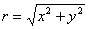. Next, suppose that the habitat independent probability of moving to any location (x,y) has a bivariate distribution,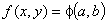, which is symmetrical about the origin.

Now consider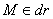, which is the event that the next move to location b, falls within an infinitesimally small annulus of width dr, with area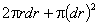, at a radius r from the starting location, a (Fig. B1). Then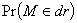is the volume of this annulus under the joint distribution f(x,y) (Pittman 1993) and because f(x,y) is symmetrical about the origin we can write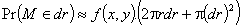. (B.1)

The probability of moving a distance r, g(r), can then be defined as (Pittman 1993)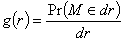,

and it follows from Eq. B.1 that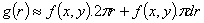and as dr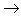0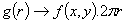. (B.2)

So, if we know g(r), i.e. the probability density function of habitat independent movement distances, then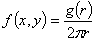. (B.3)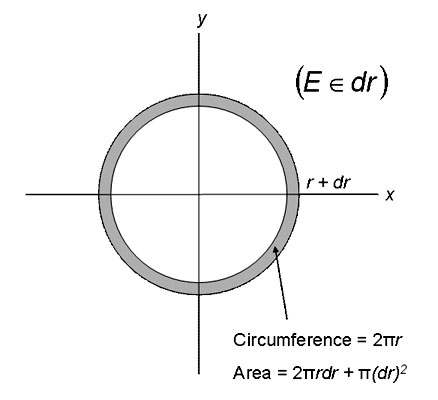FIG. B1. Diagrammatic representation of an annulus of at a radius r, with infinitesimally small width, dr. Modified from Pittman (1993).

Under the availability radius model, the habitat independent probability of moving to any location within a given radius, R, is assumed to be constant (Arthur et al. 1996). Therefore, the two-dimensional habitat independent movement probability function is defined as, (B.4)

where rab is the distance between locations a and b. We can also see, by substituting Eq. B.4 into Eq. B.3, that for this model, the probability density function of movement distances increases linearly with distance up to R, with slope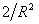.

Negative-exponential model

Under the negative-exponential model, habitat independent movement distances are assumed to have a negative-exponential distribution, with scale parameter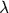. Therefore, from Eq. B.3, the two-dimensional habitat independent movement probability function is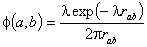. (B.5)

LITERATURE CITED

Arthur, S. M., B. F. J. Manly, L. L. McDonald, and G. W. Garner. 1996. Assessing habitat selection when availability changes. Ecology 77:215–227.

Pittman, J. 1993. Probability. Springer-Verlag, New York, New York, USA.

[Back to E086-066]